# The PANEL Procedure

### Heteroscedasticity- and Autocorrelation-Consistent Covariance Matrices

The HAC option in the MODEL statement selects the type of heteroscedasticity- and autocorrelation-consistent covariance matrix. As with the HCCME option, an estimator of the middle expression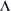in sandwich form is needed. With the HAC option, it is estimated as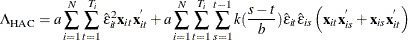, whereis the real-valued kernel function, b is the bandwidth parameter, and a is the adjustment factor of small sample degrees of freedom (that is,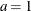if the ADJUSTDF option is not specified and otherwise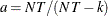, where k is the number of parameters including dummy variables). The types of kernel functions are listed in Table 20.1.

Table 20.1: Kernel Functions

Kernel Name

Equation

Bartlett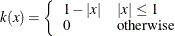Parzen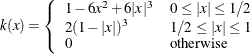Quadratic spectral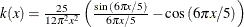Truncated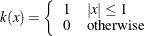Tukey-Hanning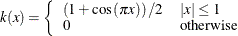When the BANDWIDTH=ANDREWS option is specified, the bandwidth parameter is estimated as shown in Table 20.2.

Table 20.2: Bandwidth Parameter Estimation

Kernel Name

Bandwidth Parameter

Bartlett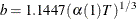Parzen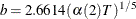Quadratic spectral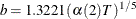Truncated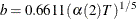Tukey-Hanning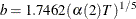Let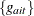denote each series in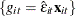, and let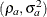denote the corresponding estimates of the autoregressive and innovation variance parameters of the AR(1) model on,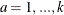, where the AR(1) model is parameterized as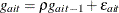with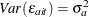. The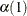and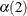are estimated with the following formulas: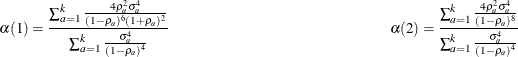When you specify BANDWIDTH=NEWEYWEST94, according to Newey and West (1994) the bandwidth parameter is estimated as shown in Table 20.3.

Table 20.3: Bandwidth Parameter Estimation

Kernel Name

Bandwidth Parameter

Bartlett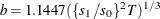Parzen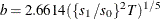Quadratic spectral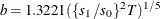Truncated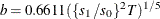Tukey-Hanning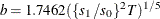The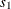and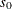are estimated with the following formulas: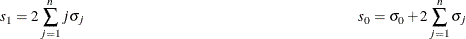where n is the lag selection parameter and is determined by kernels, as listed in Table 20.4.

Table 20.4: Lag Selection Parameter Estimation

Kernel Name

Lag Selection Parameter

Bartlett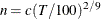Parzen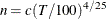Quadratic Spectral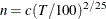Truncated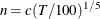Tukey-HanningThe c in Table 20.4 is specified by the C= option; by default, C=12.

The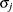is estimated with the equation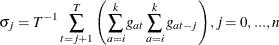where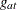is the same as in the Andrews method and i is 1 if the NOINT option in the MODEL statement is specified, and 2 otherwise.

When you specify BANDWIDTH=SAMPLESIZE, the bandwidth parameter is estimated with the equation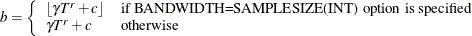where T is the sample size,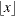is the largest integer less than or equal to x, and, r, and c are values specified by BANDWIDTH=SAMPLESIZE(GAMMA=, RATE=, CONSTANT=) options, respectively.

If the PREWHITENING option is specified in the MODEL statement,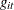is prewhitened by the VAR(1) model,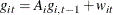Then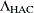is calculated by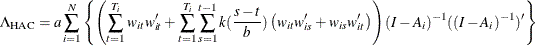The HCCME=0 with CLUSTER option sets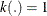.